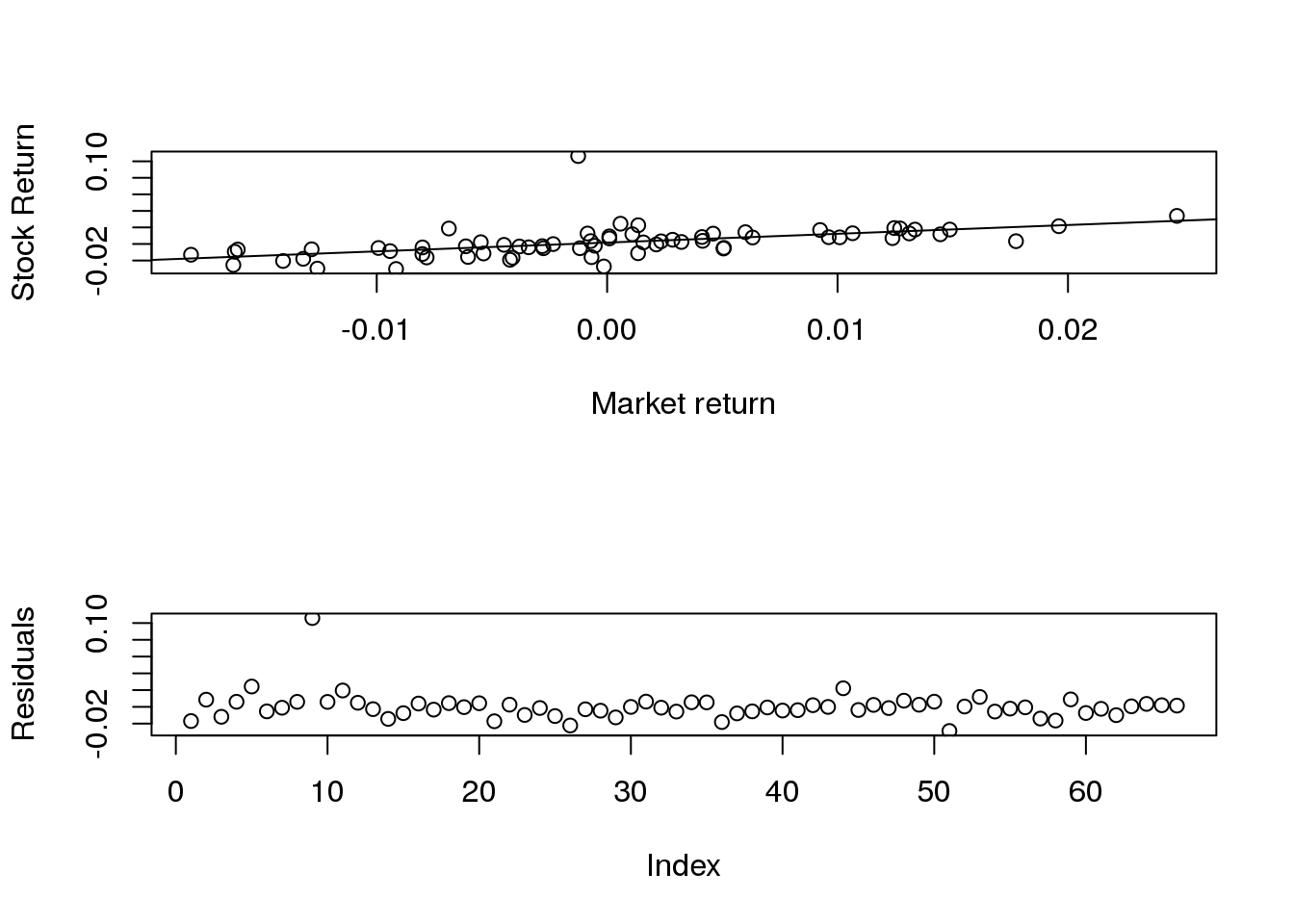Last Monday we derived the inputs to the Markowitz model from a single-factor index model. The single-factor index model assumed stock returns may be decomposed into an expected, market, and firm-specific return. We also assume returns are jointly normally distributed.

Below is an R function that will estimate the parameters of this index model. The code also creates a scatterplot of the market and stock returns and overlays the regression equation line, and then plots the regression residuals. We’ll assume the single-factor is the return on the S&P 500 index. Note, I am setting the expected return for the stock and the index to zero. The function requires we load the tseries library with

library(tseries)

Then paste the following function into the command line:

index.model <- function(z){

x <- get.hist.quote(z, start=(Sys.Date()-100), quote='Close')

x <- ts(x)

x.r <- diff(x)/x[1:(length(x)-1)]

m <- get.hist.quote("spy", start=(Sys.Date()-100), quote='Close')

m <- ts(m)

m.r <- diff(m)/m[1:(length(m)-1)]

print(summary(lm(x.r~m.r)))
par(mfrow=c(2,1))
plot(as.data.frame(x.r)[,1]~as.data.frame(m.r)[,1], xlab="Market return", ylab="Stock Return")
abline(lm(x.r~m.r))
plot(lm(x.r~m.r)\$resid, ylab="Residuals")
}

Then to use the function type the following into the command line. Note here I am estimating the index model for Red Hat, ticker: RHT. Red Hat is a linux distro vendor.

index.model("rht")
## time series starts 2014-12-08
## time series starts 2014-12-08
##
## Call:
## lm(formula = x.r ~ m.r)
##
## Residuals:
##       Min        1Q    Median        3Q       Max
## -0.028801 -0.005634 -0.000872  0.004411  0.105975
##
## Coefficients:
##             Estimate Std. Error t value Pr(>|t|)
## (Intercept) 0.001702   0.002026   0.840    0.404
## m.r         1.062636   0.217540   4.885 7.25e-06 ***
## ---
## Signif. codes:  0 '***' 0.001 '**' 0.01 '*' 0.05 '.' 0.1 ' ' 1
##
## Residual standard error: 0.01645 on 64 degrees of freedom
## Multiple R-squared:  0.2716, Adjusted R-squared:  0.2602
## F-statistic: 23.86 on 1 and 64 DF,  p-value: 7.249e-06From the output we note:

1. Alpha is insignificantly different from zero.
2. Beta is 1.06 (significantly different from zero)
3. Market returns explain 26% of the variation in RHT returns.

Try this for other stocks.

## Homework assignment:

Find an asset with a negative beta coefficient with respect to the S&P 500. Think ETF on something other than stock. Print out the results from the regression and interpret alpha, beta, and r^2 as above.